# 4.3 Fitting linear models to data  (Page 5/14)

 Page 5 / 14

A regression was run to determine whether there is a relationship between the diameter of a tree ( $\text{\hspace{0.17em}}x,$ in inches) and the tree’s age ( $\text{\hspace{0.17em}}y,$ in years). The results of the regression are given below. Use this to predict the age of a tree with diameter 10 inches.

61.966 years

For the following exercises, draw a scatter plot for the data provided. Does the data appear to be linearly related?

 0 2 4 6 8 10 –22 –19 –15 –11 –6 –2
 1 2 3 4 5 6 46 50 59 75 100 136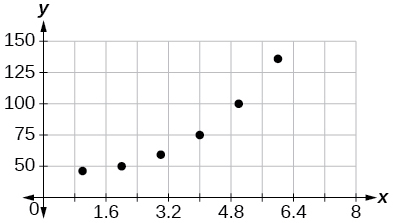No.

 100 250 300 450 600 750 12 12.6 13.1 14 14.5 15.2
 1 3 5 7 9 11 1 9 28 65 125 216No.

For the following data, draw a scatter plot. If we wanted to know when the population would reach 15,000, would the answer involve interpolation or extrapolation? Eyeball the line, and estimate the answer.

Year Population
1990 11,500
1995 12,100
2000 12,700
2005 13,000
2010 13,750

For the following data, draw a scatter plot. If we wanted to know when the temperature would reach 28°F, would the answer involve interpolation or extrapolation? Eyeball the line and estimate the answer.

 Temperature,°F 16 18 20 25 30 Time, seconds 46 50 54 55 62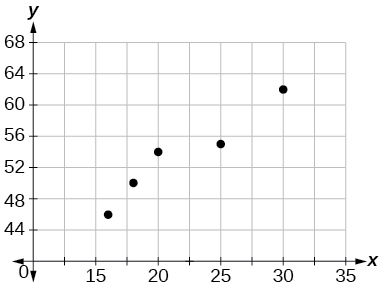Interpolation. About $\text{\hspace{0.17em}}60°F.$

## Graphical

For the following exercises, match each scatterplot with one of the four specified correlations in [link] and [link] .

$r=0.\text{95}$

$r=-0.\text{89}$

$r=-0.26$

$r=-0.39$

For the following exercises, draw a best-fit line for the plotted data.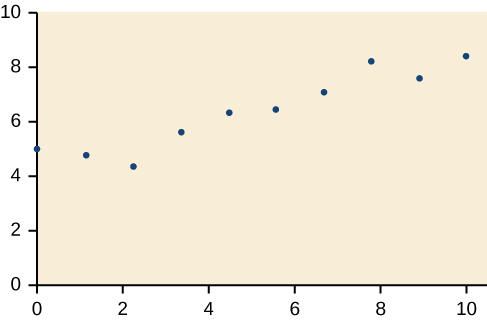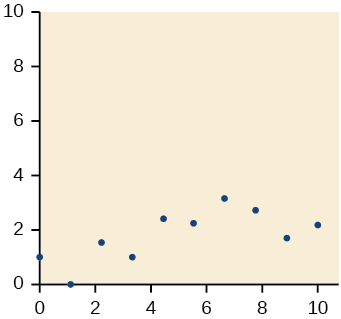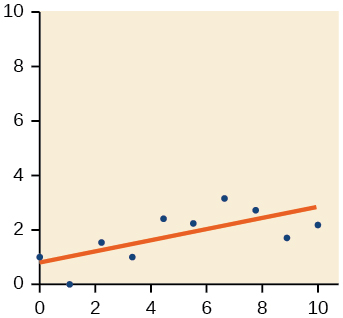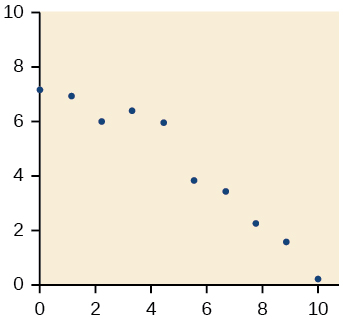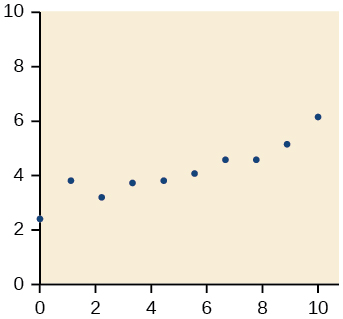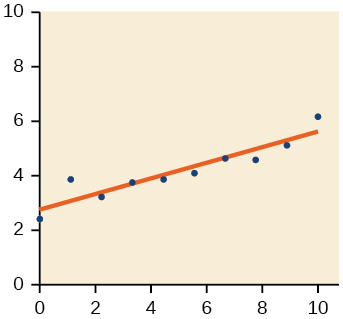## Numeric

The U.S. Census tracks the percentage of persons 25 years or older who are college graduates. That data for several years is given in [link] . Based on data from http://www.census.gov/hhes/socdemo/education/data/cps/historical/index.html. Accessed 5/1/2014. Determine whether the trend appears linear. If so, and assuming the trend continues, in what year will the percentage exceed 35%?

1990 21.3
1992 21.4
1994 22.2
1996 23.6
1998 24.4
2000 25.6
2002 26.7
2004 27.7
2006 28
2008 29.4

The U.S. import of wine (in hectoliters) for several years is given in [link] . Determine whether the trend appears linear. If so, and assuming the trend continues, in what year will imports exceed 12,000 hectoliters?

Year Imports
1992 2665
1994 2688
1996 3565
1998 4129
2000 4584
2002 5655
2004 6549
2006 7950
2008 8487
2009 9462

Yes, trend appears linear because $\text{\hspace{0.17em}}r=0.\text{985}\text{\hspace{0.17em}}$ and will exceed 12,000 near midyear, 2016, 24.6 years since 1992.

[link] shows the year and the number of people unemployed in a particular city for several years. Determine whether the trend appears linear. If so, and assuming the trend continues, in what year will the number of unemployed reach 5?

Year Number Unemployed
1990 750
1992 670
1994 650
1996 605
1998 550
2000 510
2002 460
2004 420
2006 380
2008 320

## Technology

For the following exercises, use each set of data to calculate the regression line using a calculator or other technology tool, and determine the correlation coefficient to 3 decimal places of accuracy.

 $x$ 8 15 26 31 56 $y$ 23 41 53 72 103

$y=\text{1}.\text{64}0x+\text{13}.\text{8}00,$ $r=0.\text{987}$

 $x$ 5 7 10 12 15 $y$ 4 12 17 22 24
$x$ $y$ $x$ $y$
3 21.9 10 18.54
4 22.22 11 15.76
5 22.74 12 13.68
6 22.26 13 14.1
7 20.78 14 14.02
8 17.6 15 11.94
9 16.52 16 12.76

#### Questions & Answers

write down the polynomial function with root 1/3,2,-3 with solution
if A and B are subspaces of V prove that (A+B)/B=A/(A-B)
write down the value of each of the following in surd form a)cos(-65°) b)sin(-180°)c)tan(225°)d)tan(135°)
Prove that (sinA/1-cosA - 1-cosA/sinA) (cosA/1-sinA - 1-sinA/cosA) = 4
what is the answer to dividing negative index
In a triangle ABC prove that. (b+c)cosA+(c+a)cosB+(a+b)cisC=a+b+c.
give me the waec 2019 questions
the polar co-ordinate of the point (-1, -1)
prove the identites sin x ( 1+ tan x )+ cos x ( 1+ cot x )= sec x + cosec x
tanh`(x-iy) =A+iB, find A and B
B=Ai-itan(hx-hiy)
Rukmini
what is the addition of 101011 with 101010
If those numbers are binary, it's 1010101. If they are base 10, it's 202021.
Jack
extra power 4 minus 5 x cube + 7 x square minus 5 x + 1 equal to zero
the gradient function of a curve is 2x+4 and the curve passes through point (1,4) find the equation of the curve
1+cos²A/cos²A=2cosec²A-1
test for convergence the series 1+x/2+2!/9x3ByByByBy